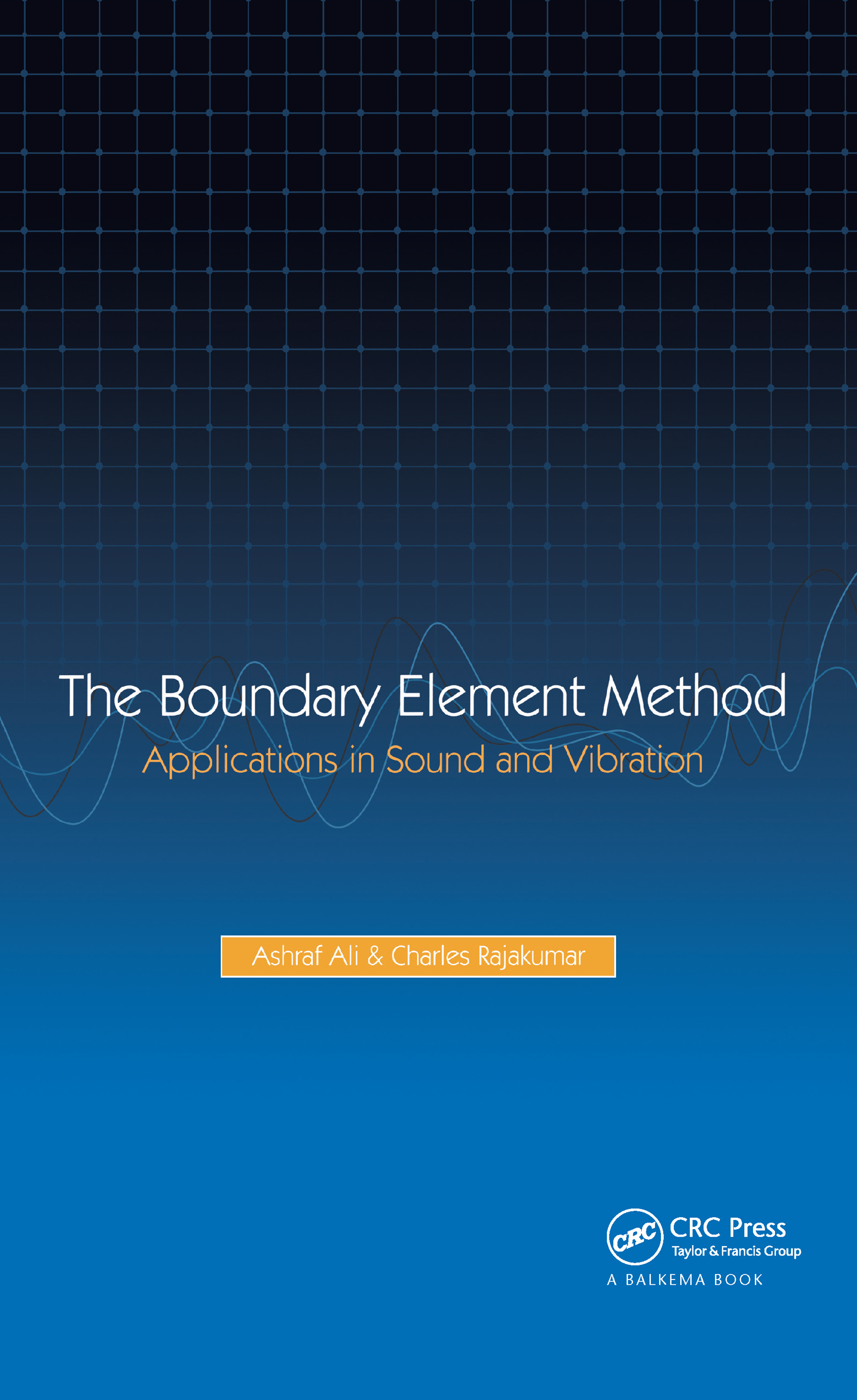The Boundary Element Method

Applications in Sound and Vibration, 1st Edition

CRC Press

200 pages

Purchasing Options:\$ = USD
Hardback: 9789058096579
pub: 2004-08-15
SAVE ~\$31.00
Currently out of stock
\$155.00
\$124.00
x
eBook (VitalSource) : 9780429077937
pub: 2004-08-15
from \$28.98

FREE Standard Shipping!

Description

The Boundary Element Method, or BEM, is a powerful numerical analysis tool with particular advantages over other analytical methods. With research in this area increasing rapidly and more uses for the method appearing, this timely book provides a full chronological review of all techniques that have been proposed so far, covering not only the fundamentals of the BEM but also a wealth of information on related computational analysis techniques and formulations, and their applications in engineering, physics and mathematics. An indispensable handbook and source of inspiration for researchers and professionals in these fields, this book is also an ideal textbook for graduate engineering students.

1 Introduction

1.1 Why the boundary element method?

1.2 Typical applications of the boundary element method

1.3 Emergence of the boundary element method

1.4 History of boundary element eigenformulations

1.5 Organization of the book

2 Boundary Element Method Fundamentals

2.1 Introduction

2.2 Direct method: weighted residuals

2.3 Examples

2.4 Direct method: Green’s integral theorem

2.5 Indirect method

2.6 Body forces

3 Isoparametric Boundary Elements

3.1 Introduction

3.2 Two-dimensional linear boundary elements

3.3 Higher-order elements in 2-D

3.4 Boundary elements in 3-D

3.5 Examples

4 Anisotropy, Axisymmetry and Zoning

4.1 Introduction

4.2 Anisotropic materials

4.3 Axisymmetric problems

4.4 Inhomogeneous regions and zoning

5 Time-Harmonic Analysis in Acoustics and Elasticity

5.1 Introduction

5.2 Acoustics

5.3 Elasticity

6 Dynamic Analysis: Acoustics and Elasticity

6.1 Introduction

6.2 Eigenvalue problem in acoustics

6.3 Eigenvalue problem in elasticity

6.4 Characteristic equation for eigenvalues

7 Basics of Algebraic Eigenvalue Problem Formulation

7.1 Introduction

7.2 Development of BE algebraic eigenvalue problem

7.3 Formulation of Internal Cell Method

7.4 Example of internal cell method: rectangular plate vibration

8 Algebraic Eigenvalue Problem in Boundary Elements

8.1 Introduction

8.2 Eigenproblem using dual reciprocity method in acoustics

8.3 Eigenproblem using particular integral method in elasticity

9 Advanced Concepts in Boundary Element Algebraic Eigenproblem

9.1 Introduction

9.2 Algebraic eigenvalue formulation using fictitious function

method

9.3 Example problems using fictitious function method

9.4 Effect of internal collocation points on eigensolutions

9.5 Polynomial-based particular integral method

9.6 Multiple reciprocity method (MRM)

9.7 Series expansion methods (SEM) with matrix augmentation

10 Acoustic Fluid–Structure Interaction Problems

10.1 Introduction

10.2 Boundary element–finite element coupled eigenanalysis of

fluid–structure system

10.3 Acoustic eigenproblem for enclosures with dissipative boundaries

10.4 Examples of acoustic eigenproblem with sound absorption

11 Solution Methods of Eigenvalue Problems

11.1 Introduction

11.2 Lanczos-based subspace approach

11.3 Lanczos recursion method

11.4 Example problems

11.5 Summary statements on the non-symmetric Lanczos eigensolver

11.6 Damped system eigenvalue problem solution

11.7 Lanczos two-sided recursion for the quadratic eigenvalue problem

11.8 Summary statements on eigenvalue computation algorithms

12 Discussion and Future Research

12.1 Discussion on boundary element eigenvalue methodologies

12.2 Comparison of eigenanalysis using BEM and FEM

12.3 Topics not covered in the book

12.4 Future research on BEM eigenanalysis

Ali\, A.; Rajakumar\, C.